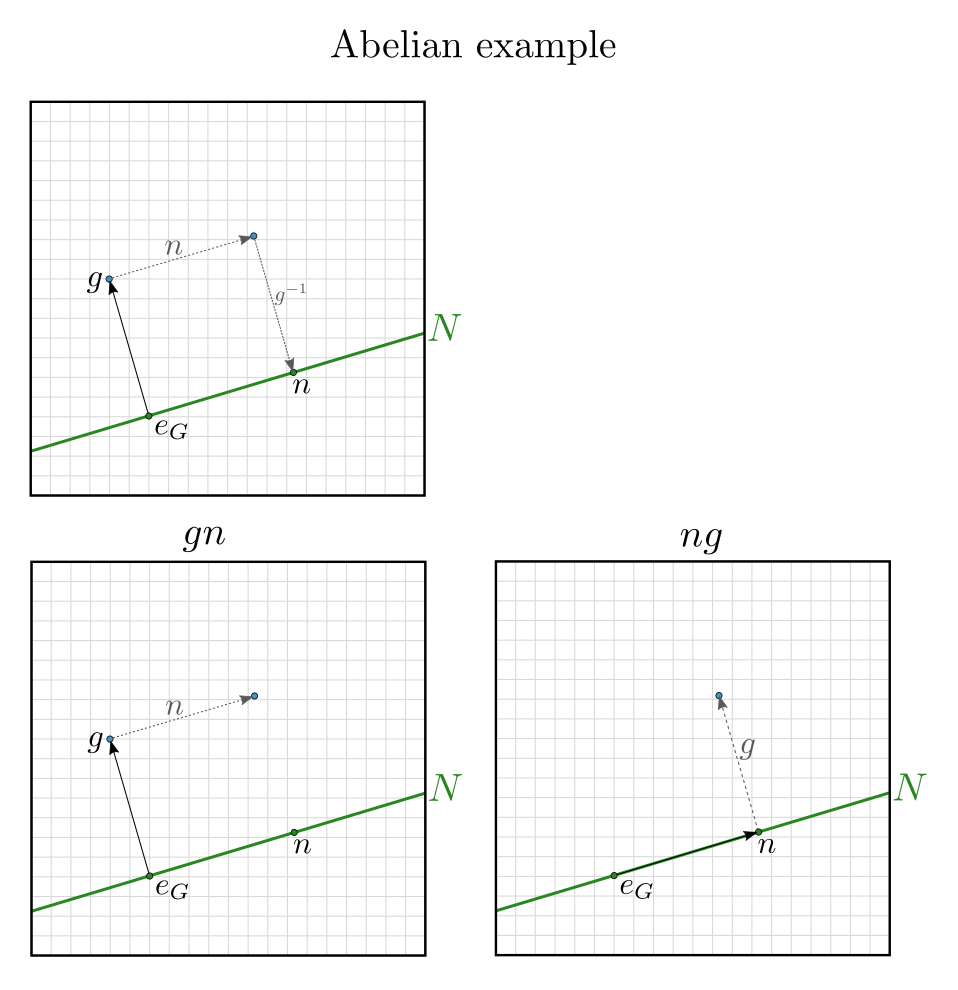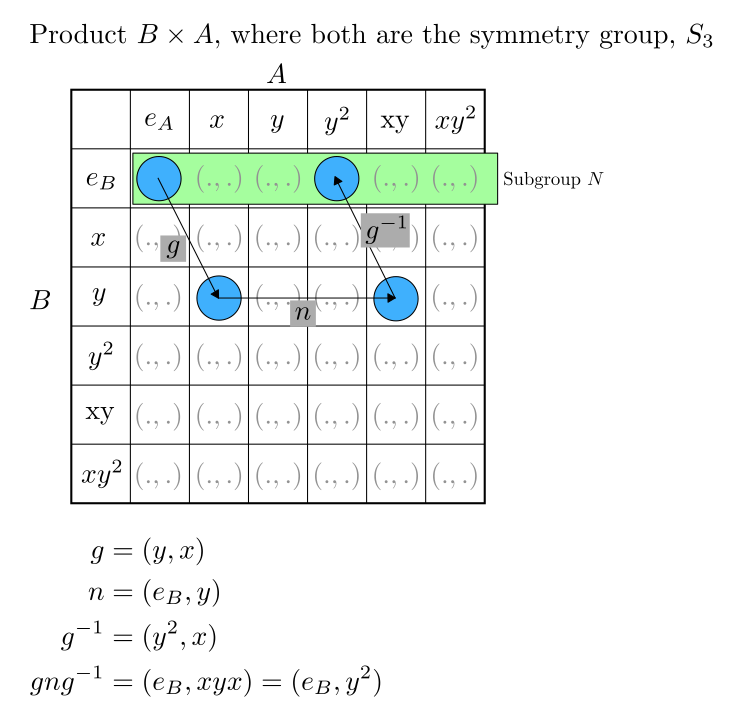Show Question
Math and science::Algebra::Aluffi

# Normal subgroup

### Normal subgroup

Let $$G$$ be a group and $$N$$ a subgroup of $$G$$.

$$N$$ is said to be normal iff:

$\forall n \in N, \forall g \in G, \; gng^{-1} \in N$

Or equivalently:

$N \text{ is normal } \iff \forall g \in G, \; gN = Ng$

$$gN$$ is syntax for $$\{ a : a = gn \text{ for some } n \in N \}$$, and $$gN = Ng$$ does not imply commutativity.

### Abelian normal subgroupNote how in the Abelian case, $$gng^{-1} = n$$, which is a stronger result than $$gng^{-1} \in N$$.

### Non-abelian normal subgroup

Products have non-abelian normal subgroups.